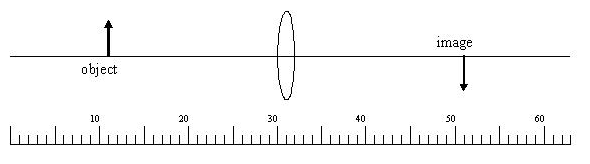# Problem: A. An object is 1.0 cm tall and its erect image is 4.0 cm tall. What is the exact magnification?B. When using the Lens Equation, a virtual image has aa. positive object distanceb. negative image distanceC. The scale at the bottom of the diagram is calibrated in cm. Find the focal length of the lens (in cm). (Do not include units with the answer.)

###### FREE Expert Solution

This problem involves the formation of images using lenses.

A.

Magnification:

$\overline{){\mathbf{m}}{\mathbf{=}}\frac{{\mathbf{h}}_{\mathbf{i}}}{{\mathbf{h}}_{\mathbf{o}}}{\mathbf{=}}{\mathbf{-}}\frac{{\mathbf{s}}_{\mathbf{i}}}{{\mathbf{s}}_{\mathbf{o}}}}$

The image is upright, hi = +4.0cm

90% (114 ratings)###### Problem Details

A. An object is 1.0 cm tall and its erect image is 4.0 cm tall. What is the exact magnification?

B. When using the Lens Equation, a virtual image has a

a. positive object distance
b. negative image distance

C. The scale at the bottom of the diagram is calibrated in cm. Find the focal length of the lens (in cm). (Do not include units with the answer.)